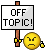# GuiCtrlCreateInput hellp

Go to solution Solved by Subz,

## Recommended Posts

hi, all.

i create a gui form use GuiCtrlCreateInput() to get user input data but GuiCtrlCreateInput() return control id.

i use msgbox(0, "", guictrlread(guictrlcreateinput()) that can display user input data.

but i want to put the user date into an array, guictrlcrateinput() return blank,

i try to use _filereadtoarray(), but user data is not a file, only some data, such as 1, 2, a, b,etc.

is there a function like _DateReadToArray() ?

thank you all.

##### Share on other sites

thank you all.

i use _IsPressed to get keyboard event, when users key in some date then press TAB key.

i use controlgettxt to get its data.

##### Share on other sites
• Solution

You would need to update your array when the user clicks OK

Example:

```Case \$Button1
MsgBox(0,"Msgbox",\$TestArray1)```

##### Share on other sitesdirect from AutoIt help

```#include <MsgBoxConstants.au3>

Example()

Func Example()
; Sample script using @NumParams macro
Test_Numparams(1, 2, 3, 4, 5, 6, 7, 8, 9, 10, 11, 12, 13, 14)
EndFunc   ;==>Example

Func Test_Numparams(\$vVar1 = 0, \$vVar2 = 0, \$vVar3 = 0, \$vVar4 = 0, \$vVar5 = 0, \$vVar6 = 0, \$vVar7 = 0, \$vVar8 = 0, \$vVar9 = 0, _
\$vVar10 = 0, \$vVar11 = 0, \$vVar12 = 0, \$vVar13 = 0, \$vVar14 = 0, \$vVar15 = 0, \$vVar16 = 0, \$vVar17 = 0, \$vVar18 = 0, \$vVar19 = 0)
#forceref \$vVar1, \$vVar2, \$vVar3, \$vVar4, \$vVar5, \$vVar6, \$vVar7, \$vVar8, \$vVar9, \$vVar10
#forceref \$vVar11, \$vVar12, \$vVar13, \$vVar14, \$vVar15, \$vVar16, \$vVar17, \$vVar18, \$vVar19
Local \$sVal = ""
For \$i = 1 To @NumParams
\$sVal &= Eval("vVar" & \$i) & " "
Next
MsgBox(\$MB_SYSTEMMODAL, "", "@NumParams = " & @NumParams & @CRLF & @CRLF & \$sVal)
EndFunc   ;==>Test_Numparams```

Eval ( string )

Edited by ioa747
##### Share on other sites
20 hours ago, Subz said:

You would need to update your array when the user clicks OK

Example:

```Case \$Button1
MsgBox(0,"Msgbox",\$TestArray1)```

thank you , your way simplifies my code.

##### Share on other sites
13 hours ago, ioa747 said:direct from AutoIt help

```#include <MsgBoxConstants.au3>

Example()

Func Example()
; Sample script using @NumParams macro
Test_Numparams(1, 2, 3, 4, 5, 6, 7, 8, 9, 10, 11, 12, 13, 14)
EndFunc   ;==>Example

Func Test_Numparams(\$vVar1 = 0, \$vVar2 = 0, \$vVar3 = 0, \$vVar4 = 0, \$vVar5 = 0, \$vVar6 = 0, \$vVar7 = 0, \$vVar8 = 0, \$vVar9 = 0, _
\$vVar10 = 0, \$vVar11 = 0, \$vVar12 = 0, \$vVar13 = 0, \$vVar14 = 0, \$vVar15 = 0, \$vVar16 = 0, \$vVar17 = 0, \$vVar18 = 0, \$vVar19 = 0)
#forceref \$vVar1, \$vVar2, \$vVar3, \$vVar4, \$vVar5, \$vVar6, \$vVar7, \$vVar8, \$vVar9, \$vVar10
#forceref \$vVar11, \$vVar12, \$vVar13, \$vVar14, \$vVar15, \$vVar16, \$vVar17, \$vVar18, \$vVar19
Local \$sVal = ""
For \$i = 1 To @NumParams
\$sVal &= Eval("vVar" & \$i) & " "
Next
MsgBox(\$MB_SYSTEMMODAL, "", "@NumParams = " & @NumParams & @CRLF & @CRLF & \$sVal)
EndFunc   ;==>Test_Numparams```

Eval ( string )

a little complexity, i do not understand so much.

but thank you.

## Create an account

Register a new account

• ### Recently Browsing   0 members

×

• Wiki

• Back

• #### Beta

• Git
• FAQ
×
• Create New...